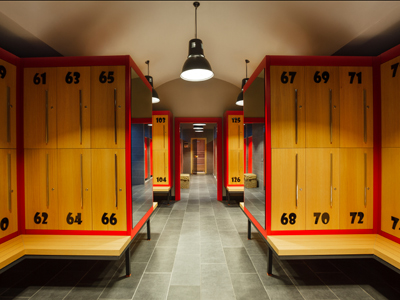Keep playing this quiz until you get 10 out of 10!

# Pre-Algebra - Monomials and Polynomials: Multiplying

This Math quiz is called 'Pre-Algebra - Monomials and Polynomials: Multiplying' and it has been written by teachers to help you if you are studying the subject at middle school. Playing educational quizzes is a fabulous way to learn if you are in the 6th, 7th or 8th grade - aged 11 to 14.

It costs only \$12.50 per month to play this quiz and over 3,500 others that help you with your school work. You can subscribe on the page at Join Us

As you have previous learned, a monomial is one number that stands on its own and a polynomial is two or more monomial numbers that are linked together in an equation by an addition sign (+), subtraction sign (-) or a multiplication sign (x). For example: 3x2 ● 5x5. The “●” symbol will be used here in place of the normal “x” used for multiplication so as to not confuse “x” with “x” in algebra problems.

When multiplying monomials, any exponents are added together. So in the example 3x2 ● 5x5, “2” and “5” will be added and the equations will then look like:

3x2 ● 5x5
3 ● 5 = 15x7

When multiplying polynomials, any exponents are added. Let’s look at the following example: (3x3)(4x4). [Note: This is a polynomial. When the “●” is replaced with parentheses(), the numbered problem becomes a polynomial and the parentheses indicate that we will be multiplying the two monomial numbers.] This problem is worked as follows to find the solution.

(3x3)(4x4)

3 ● 4 = 12
x3 + x4 = x7
Solution: 12x7

Now let’s look at the next example of 2x(3x + 7) and see how to find the solution.

2x(3x + 7)
(2x)(3x) + (2x)(7)
6x2 + 14x

1.
7x(4x2 + 3x + 12)
28x3 + 21x2 - 84x
28x3 + 21x + 84x
11x3 + 10x2 + 19x
28x3 + 21x2 + 84x
7x(4x2 + 3x + 12)
(7x)(12) = 84x
Solution: 28x3 + 21x2 + 84x
Answer (d) is the correct solution
2.
12x4 ● 10x2
120x6
102x8
120x8
22x6
12x4 ● 10x2
12 ● 10 = 120x6 (remember to add exponents)
Solution: 120x6
Answer (a) is the correct solution
3.
(8x5)(3x2)
24x10
11x7
24x7
11x10
(8x5)(3x2)
8 ● 3 = 24
x5 + x2 = x7
Solution: 24x7
Answer (c) is the correct solution
4.
6x5 ● 3x4
18x20
18x9
9x9
9x20
6x5 ● 3x4
6 ● 3 = 18x9 (remember to add exponents)
Solution: 18x9
Answer (b) is the correct solution
5.
x(5x2 - 2x + 3)
20x3 - 8x2 + 12x
20x2 - 8x + 12x
9x3 - 2x2 + 7x
9x3 - 8x2 + 12x
4x(5x2 - 2x + 3)
(4x)(3) = 12x
Solution: 20x3 - 8x2 + 12x
Answer (a) is the correct solution
6.
10x(5x2 - 9x + 22)
50x3 + 90x2 + 220x
50x3 - 90x + 220x
50x3 - 90x2 + 220x
50x3 - 90x2 + 120x
10x(5x2 - 9x + 22)
(10x)(22) = 220x
Solution: 50x3 - 90x2 + 220x
Answer (c) is the correct solution
7.
x(20x2 + 10x + 100)
20x3 + 10x + 100x2
20x2 + 10x + 100x
20x3 + 10x2 + 100x
20x2 + 10x2 + 100x
x(20x2 + 10x + 100)
(x)(100) = 100x
Solution: 20x3 + 10x2 + 100x
Answer (c) is the correct solution
8.
3x(17x4 + 5x2 + 13)
51x5 + 15x + 39x2
51x8 + 15x2 + 39x2
51x4 + 15x2 + 39x
51x5 + 15x3 + 39x
3x(17x4 + 5x2 + 13)
(3x)(13) = 39x
Solution: 51x5 + 15x3 + 39x
Answer (d) is the correct solution
9.
(21x9)(8x3)
168x27
168x12
168x6
168x9
(21x9)(8x3)
21 ● 8 = 168
x9 + x3 = x12
Solution: 168x12
Answer (b) is the correct solution
10.
15x2 ● 5x8
75x10
20x10
20x16
75x16
15x2 ● 5x8
15 ● 5 = 75x10 (remember to add exponents)
Solution: 75x10
Answer (a) is the correct solution
Author:  Christine G. Broome